Function Repository Resource:

# ToNegabinary

Get the negabinary representation of an integer

Contributed by: Eric W. Weisstein
 ResourceFunction["ToNegabinary"][n] gives the negabinary representation of the integer n.

## Details

Negabinary is a representation of an integer in base –2.

## Examples

### Basic Examples (4)

Get the representation for 17 in negabinary:

 In:=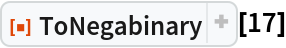Out=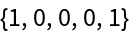From this representation, recover the original integer:

 In:=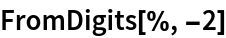Out=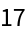Use the resource function FromNegabinary:

 In:=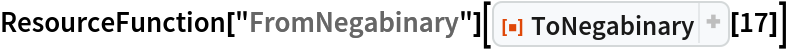Out=Some integers have the same representation in base 2:

 In:=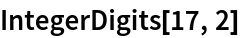Out=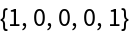### Scope (1)

ToNegabinary handles negative numbers:

 In:=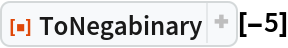Out=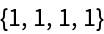### Applications (2)

Plot the negabinary representation for the first 22 integers:

 In:=Out=A table of integers and their negabinary representations:

 In:=Out=### Properties and Relations (1)

Numbers belonging to the Moser–de Bruijn sequence have identical binary and negabinary representations:

 In:=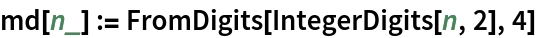In:=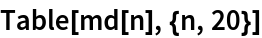Out=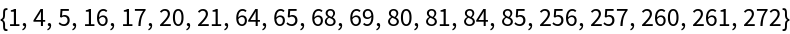In:=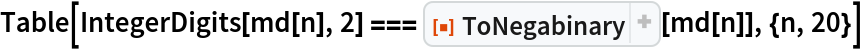Out=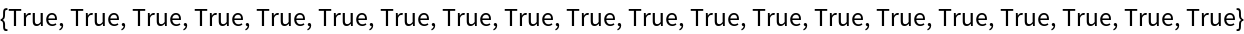## Requirements

Wolfram Language 11.3 (March 2018) or above

## Version History

• 1.0.1 – 31 August 2021
• 1.0.0 – 11 February 2019